Date: Mon, 13 Jul 1998 13:43:32 -0600 (CST)
Subject: high school algebra

Name: Fred
Who is asking: Student
Level: Secondary

Question:
I need a step by step lesson on solving quadratic equations.

Thank you,

Fred for my daughter

Hi Fred,
If your daughter is solving quadratics then she has already learned about factoring. If the quadratic can be factored then this is the easiest way to solve the quadratic. For example if you are to solve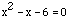then, factoring the quadratic gives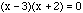Thus x=3 or x=-2.

If the quadratic does not factor then you can use the "general quadratic" which says that if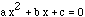then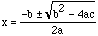.

For example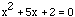has solution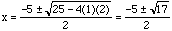.

Cheers
Penny

Go to Math Central

To return to the previous page use your browser's back button.

Math Central